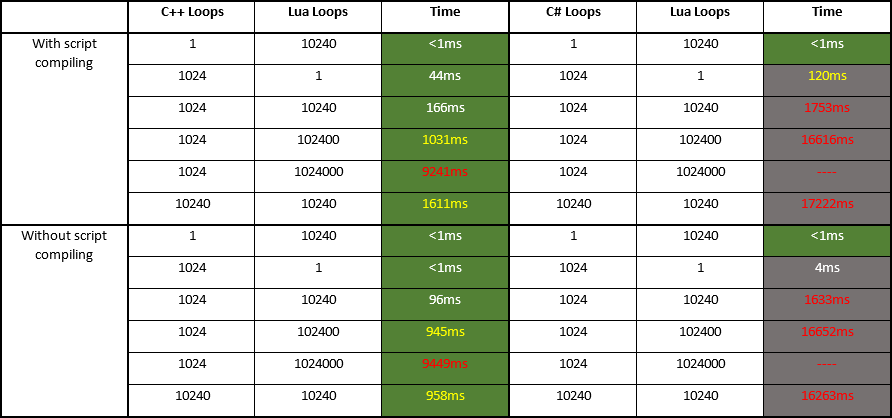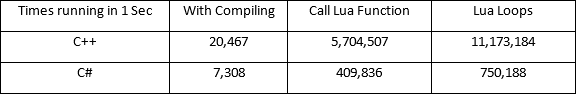C#的Lua我们用的是云风团队出品的UniLua，对C#的Lua库了解不太多就选用了这个还算比较流行的库，C++版本是标准的Lua但要提前说明的是我们进行了修改，这是个去掉了浮点数运算的特别版本，不过测试的目的仅仅是为了得出大致的性能差距，并不是用来做学术研究，所以测试的方法非常简单，相信得到的结果也并不那么科学严谨，只能是在特定的前提条件范围内的粗略结果，较真儿的朋友们请出门儿右转找政府。

``````SYSTEMTIME time;
GetSystemTime(&time);
// printf("%d, %d,n", time.wSecond, time.wMilliseconds);
int startTime = time.wSecond * 1000 + time.wMilliseconds;
for(int i = 0; i < 1024; i++) {
lua_getglobal(g_lua_state_instance, "measureINT");
int result = lua_pcall(g_lua_state_instance, 0, 0, 0);
}
GetSystemTime(&time);
// printf("%d, %d,n", time.wSecond, time.wMilliseconds);
int gapTime = time.wSecond * 1000 + time.wMilliseconds - startTime;
if (gapTime < 0) { gapTime += 60000; }
printf("Used %d millisecondsn", gapTime);
``````
``````function measureINT()
num = 1024
for i=1, num do
local a = 1
local b = 1
local c = a + b
local d = c + 5
end
end
``````